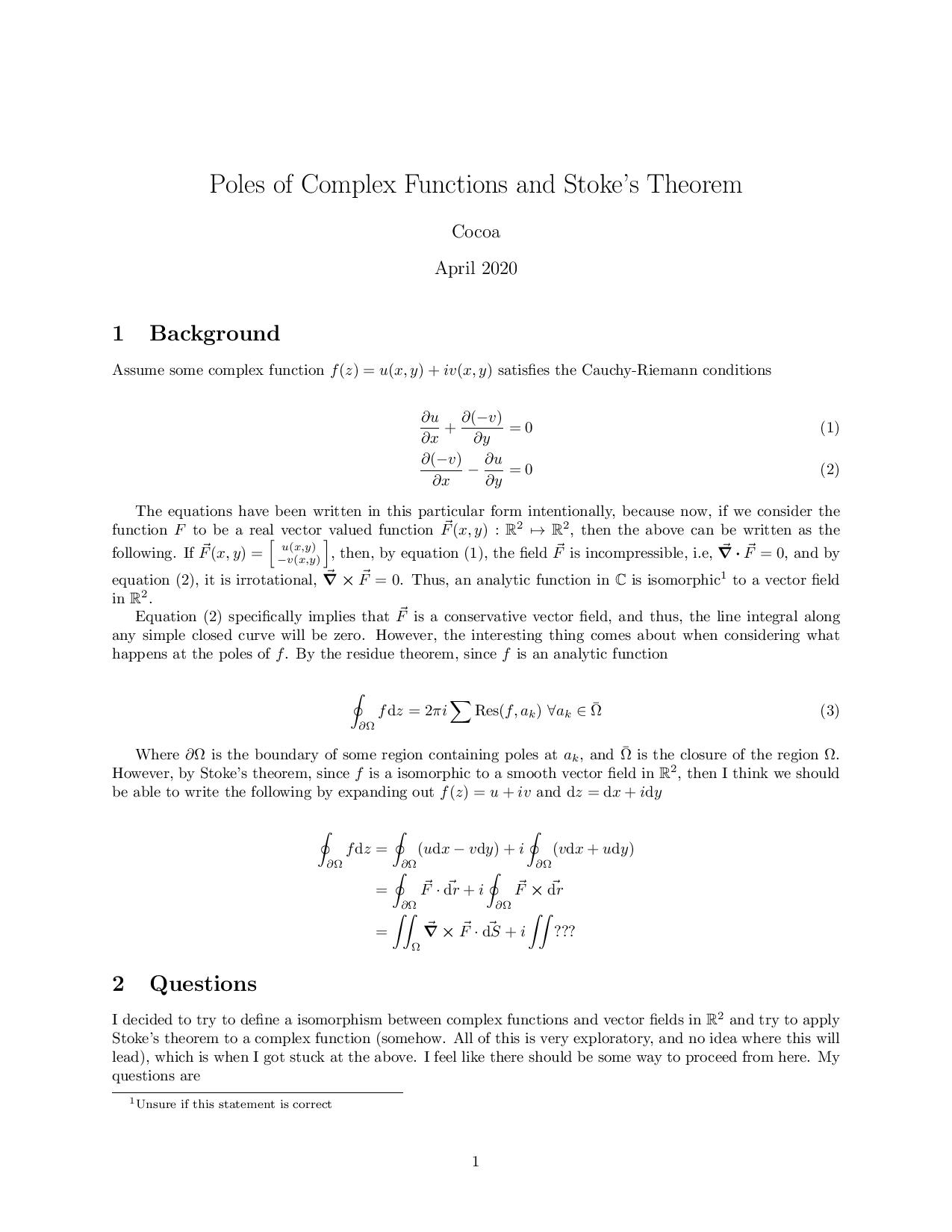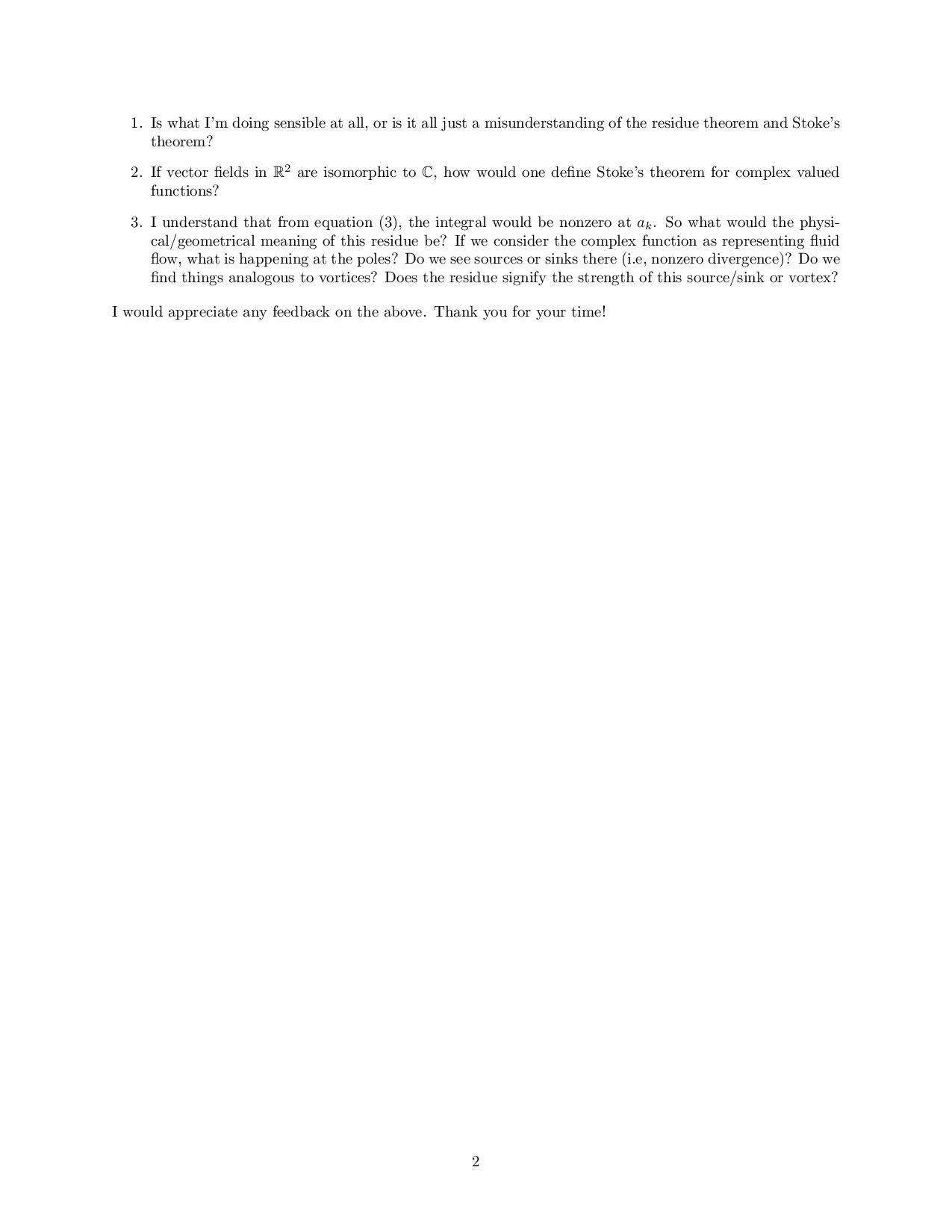# Is the complex plan isomorphic to R2?

A complex number $a+bi$ can be represented as an element of the manifold $\mathbb{R}^2$ with the point $(a,b)$ (as that's how I've learned about Complex Numbers and thought of the complex plane). Thus we have a natural isomorphism between complex numbers $\mathbb{C}$ and the real plane $\mathbb{R^2}$.

If we continue, we can now think of an isomorphism between the set of analytic functions on the complex plane $f:\mathbb{C}\mapsto\mathbb{C}$ to vector fields on the real plane $\vec F : \mathbb{R}^2 \mapsto \mathbb{R}^2$. However, I tried to apply Stoke's theorem around a closed loop at the pole of an analytic complex function to get something like the Residue theorem, however I ran into an issue from which I did not know how to proceed. I've attached two images explaining my thought process.All this led me to realize that you can divide complex numbers but you can't divide elements of $\mathbb{R}^2$ with each other. So I have the following question in addition to the questions mentioned in the image - Is the usual topology of the complex plane the same as the usual open ball topology of $\mathbb{R}^2$? If not, can we accurately draw analogies between complex numbers and the real plane?Note by Cocoa Butter
11 months ago

This discussion board is a place to discuss our Daily Challenges and the math and science related to those challenges. Explanations are more than just a solution — they should explain the steps and thinking strategies that you used to obtain the solution. Comments should further the discussion of math and science.

When posting on Brilliant:

• Use the emojis to react to an explanation, whether you're congratulating a job well done , or just really confused .
• Ask specific questions about the challenge or the steps in somebody's explanation. Well-posed questions can add a lot to the discussion, but posting "I don't understand!" doesn't help anyone.
• Try to contribute something new to the discussion, whether it is an extension, generalization or other idea related to the challenge.
• Stay on topic — we're all here to learn more about math and science, not to hear about your favorite get-rich-quick scheme or current world events.

MarkdownAppears as
*italics* or _italics_ italics
**bold** or __bold__ bold
- bulleted- list
• bulleted
• list
1. numbered2. list
1. numbered
2. list
Note: you must add a full line of space before and after lists for them to show up correctly
paragraph 1paragraph 2

paragraph 1

paragraph 2

[example link](https://brilliant.org)example link
> This is a quote
This is a quote
    # I indented these lines
# 4 spaces, and now they show
# up as a code block.

print "hello world"
# I indented these lines
# 4 spaces, and now they show
# up as a code block.

print "hello world"
MathAppears as
Remember to wrap math in $$ ... $$ or $ ... $ to ensure proper formatting.
2 \times 3 $2 \times 3$
2^{34} $2^{34}$
a_{i-1} $a_{i-1}$
\frac{2}{3} $\frac{2}{3}$
\sqrt{2} $\sqrt{2}$
\sum_{i=1}^3 $\sum_{i=1}^3$
\sin \theta $\sin \theta$
\boxed{123} $\boxed{123}$

## Comments

There are no comments in this discussion.

×

Problem Loading...

Note Loading...

Set Loading...